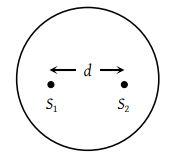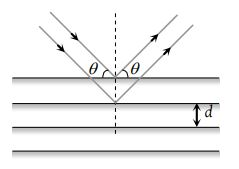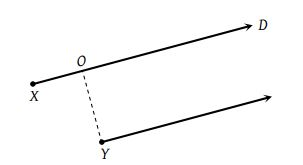In a YDSE bi-chromatic light of wavelengths, 400 nm and 560 nm are used. The distance between the slits is 0.1 mm and the distance between the plane of the slits and the screen is 1m. The minimum distance between two successive regions of complete darkness is

(1) 4 mm

(2) 5.6 mm

(3) 14 mm

(4) 28 mm

Concept Questions :-

Young Double slit experiment
High Yielding Test Series + Question Bank - NEET 2020

Difficulty Level:

In Young's double-slit experiment, the intensity at a point is (1/4) of the maximum intensity. Angular position of this point is :

(1) sin-1(λ/d)

(2) sin-1(λ/2d)

(3) sin-1(λ/3d)

(4) sin-1(λ/4d)

Concept Questions :-

Young Double slit experiment
High Yielding Test Series + Question Bank - NEET 2020

Difficulty Level:

A beam of electron is used in a YDSE experiment. The slit width is d. When the velocity of the electron is increased, then,

(1) No interference is observed

(2) Fringe width increases

(3) Fringe width decreases

(4) Fringe width remains the same

Concept Questions :-

Young Double slit experiment
High Yielding Test Series + Question Bank - NEET 2020

Difficulty Level:

If the separation between screen and source is increased by 2% what would be the effect on the intensity :

(1) Increases by 4%

(2) Increases by 2%

(3) Decreases by 2%

(4) Decreases by 4%

Concept Questions :-

Young Double slit experiment
High Yielding Test Series + Question Bank - NEET 2020

Difficulty Level:

The ratio of intensities of consecutive maxima in the diffraction pattern due to a single slit is

(1) 1 : 4 : 9

(2) 1 : 2 : 3

(3) $1\text{\hspace{0.17em}}:\frac{4}{9\text{\hspace{0.17em}}{\pi }^{2}}:\frac{4}{25\text{\hspace{0.17em}}{\pi }^{2}}$

(4) $1:\frac{1}{{\pi }^{2}}:\frac{9}{{\pi }^{2}}$

Concept Questions :-

Diffraction
High Yielding Test Series + Question Bank - NEET 2020

Difficulty Level:

In a single slit diffraction of light of wavelength λ by a slit of width e, the size of the central maximum on a screen at a distance b is

(1) $2b\lambda +e$

(2) $\frac{2b\lambda }{e}$

(3) $\frac{2b\lambda }{e}+e$

(4) $\frac{2b\lambda }{e}-e$

Concept Questions :-

Diffraction
High Yielding Test Series + Question Bank - NEET 2020

Difficulty Level:

A circular disc is placed in front of a narrow source. When the point of observation is 2 m from the disc, then it covers first HPZ. The intensity at this point is I. When the point of observation is 25 cm from the disc then intensity will be

(1) ${\left(\frac{{R}_{6}}{{R}_{2}}\right)}^{2}I$

(2) ${\left(\frac{{R}_{7}}{{R}_{2}}\right)}^{2}I$

(3) ${\left(\frac{{R}_{8}}{{R}_{2}}\right)}^{2}I$

(4) ${\left(\frac{{R}_{9}}{{R}_{2}}\right)}^{2}I$

Concept Questions :-

Interference vs Diffraction
High Yielding Test Series + Question Bank - NEET 2020

Difficulty Level:

Two coherent sources separated by distance d are radiating in phase having wavelength λ. A detector moves in a big circle around the two sources in the plane of the two sources. The angular position of n = 4 interference maxima is given as(1) ${\mathrm{sin}}^{-1}\frac{n\lambda }{d}$

(2) ${\mathrm{cos}}^{-1}\frac{4\lambda }{d}$

(3) ${\mathrm{tan}}^{-1}\frac{d}{4\lambda }$

(4) ${\mathrm{cos}}^{-1}\frac{\lambda }{4d}$

Concept Questions :-

Interference vs Diffraction
High Yielding Test Series + Question Bank - NEET 2020

Difficulty Level:

A beam with wavelength λ falls on a stack of partially reflecting planes with separation d. The angle θ that the beam should make with the planes so that the beams reflected from successive planes may interfere constructively is (where n =1, 2, ……)(1) ${\mathrm{sin}}^{-1}\left(\frac{n\lambda }{d}\right)$

(2) ${\mathrm{tan}}^{-1}\left(\frac{n\lambda }{d}\right)$

(3) ${\mathrm{sin}}^{-1}\left(\frac{n\lambda }{2d}\right)$

(4) ${\mathrm{cos}}^{-1}\left(\frac{n\lambda }{2d}\right)$

Concept Questions :-

Interference vs Diffraction
High Yielding Test Series + Question Bank - NEET 2020

Difficulty Level:

Two point sources X and Y emit waves of same frequency and speed but Y lags in phase behind X by 2πl radian. If there is a maximum in direction D the distance XO using n as an integer is given by(1) $\frac{\lambda }{2}\left(n-l\right)$

(2) $\lambda \left(n+l\right)$

(3) $\frac{\lambda }{2}\left(n+l\right)$

(4) $\lambda \left(n-l\right)$

Concept Questions :-

Diffraction